# Maximum and minimum points

Points in the domain of definition of a real-valued function at which it takes its greatest and smallest values; such points are also called absolute maximum and absolute minimum points. Ifis defined on a topological space, then a pointis called a local maximum (local minimum) point if there is a neighbourhood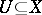ofsuch thatis an absolute maximum (minimum) point for the restriction ofto this neighbourhood. One distinguishes between strict and non-strict maximum (minimum) points (both absolute and local). For example, a point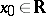is called a non-strict (strict) local maximum point ofif there is a neighbourhoodofsuch that for all,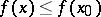(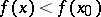,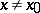).
For functions defined on finite-dimensional domains there are conditions and tests, in terms of differential calculus, for a given point to be a local maximum (minimum) point. Letbe defined in a neighbourhood of a pointof the real line. Ifis a non-strict local maximum (minimum) point and if the derivative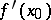exists, then the latter is equal to zero.
If a functionis differentiable in a neighbourhood ofexcept, possibly, atitself where it is continuous, and if the derivativeis of constant sign on each side ofin this neighbourhood, then forto be a strict local maximum (local minimum) point it is necessary and sufficient that the derivative changes sign from plus to minus, that is,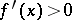forand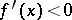for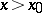(respectively, from minus to plus;for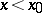andfor). However, it is not possible to speak of the change of sign of the derivative atfor every functionthat is differentiable in a neighbourhood of.
Ifhasderivatives atand if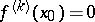,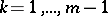,, then forto be a strict local maximum point it is necessary and sufficient thatbe even and that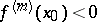, and for a local minimum thatbe even and.
Let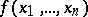be defined in an-dimensional neighbourhood of a point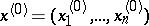and let it be differentiable at this point. Ifis a non-strict local maximum (minimum) point, then the differential ofat this point is equal to zero. This condition is equivalent to all first-order partial derivatives ofbeing zero at this point. If the function has continuous second-order partial derivatives at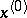, if all its first-order derivatives are equal to zero at, and if the second-order differential atis a negative-definite (positive-definite) quadratic form, thenis a strict local maximum (minimum) point. Conditions for maximum and minimum points of differentiable functions are known when restrictions are imposed on the variation of the arguments in the domain: coupling equations must be satisfied. Necessary and sufficient conditions for a maximum (minimum) of a real-valued function with a more complicated structure of its domain of definition have been obtained in special areas of mathematics; for example, in convex analysis and mathematical programming (see also Maximization and minimization of functions). Maximum and minimum points of functions on manifolds are studied in variational calculus in the large, and maximum and minimum points for functions on function spaces, that is, for functionals, are studied in variational calculus. There are also various numerical approximation methods for finding maximum and minimum points.